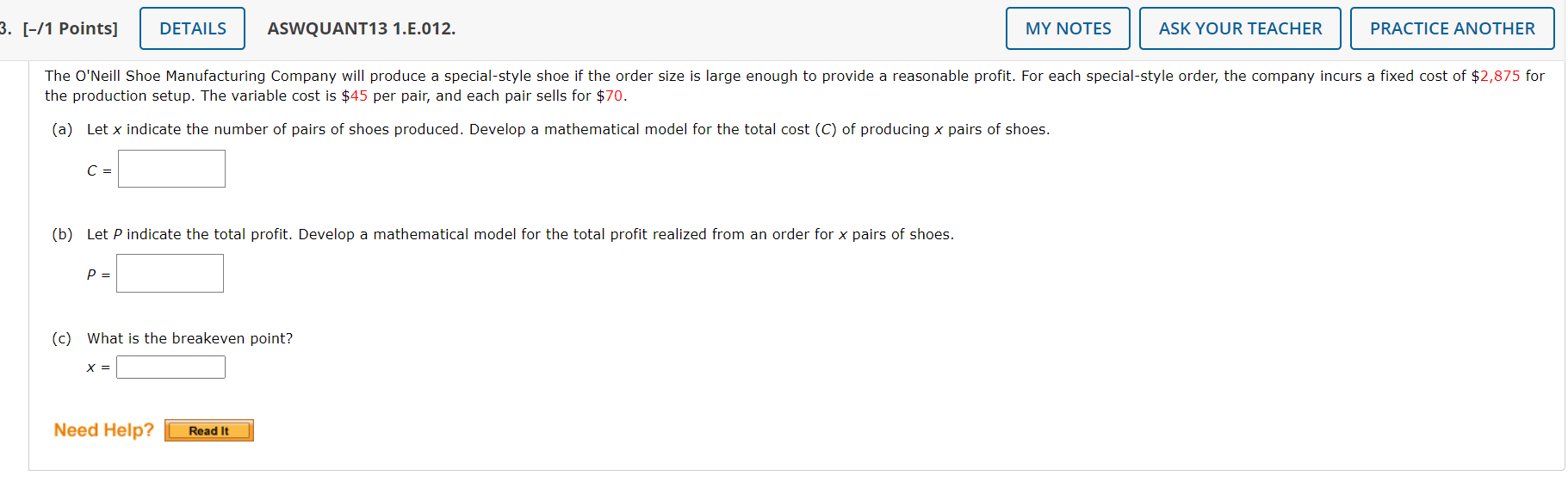Home / Expert Answers / Statistics and Probability / 1-points-aswquant13-1-e-012-the-production-setup-the-variable-cost-is-45-per-pair-pa624

# (Solved): [-/1 Points] ASWQUANT13 1.E.012. the production setup. The variable cost is \( \\$ 45 \) per pair, ...[-/1 Points] ASWQUANT13 1.E.012. the production setup. The variable cost is \( \\$ 45 \) per pair, and each pair sells for \( \\$ 70 \). (a) Let \( x \) indicate the number of pairs of shoes produced. Develop a mathematical model for the total cost \( (C) \) of producing \( x \) pairs of shoes. \( C= \) (b) Let \( P \) indicate the total profit. Develop a mathematical model for the total profit realized from an order for \( x \) pairs of shoes. \( P= \) (c) What is the breakeven point? \( x= \)

We have an Answer from Expert